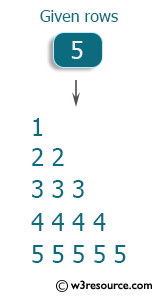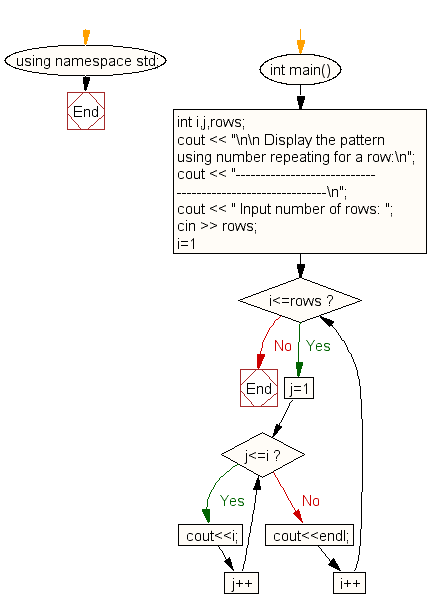﻿ C++ : Pattern like right angle triangle with a number

# C++ Exercises: Display the pattern like right angle triangle using number which will repeat the number for that row

## C++ For Loop: Exercise-37 with Solution

Write a C++ program that makes a pattern such as a right angle triangle using numbers that repeat.

Visual Presentation:Sample Solution:

C++ Code :

``````#include <iostream> // Include the input/output stream library
using namespace std; // Using standard namespace

int main() // Main function where the execution of the program starts
{
int i, j, rows; // Declare integer variables i, j, and rows

// Display message asking for input
cout << "\n\n Display the pattern using number repeating for a row:\n";
cout << "----------------------------------------------------------\n";
cout << " Input number of rows: ";
cin >> rows; // Read input for the number of rows from the user

// Loop to print the pattern using repeated numbers for each row
for (i = 1; i <= rows; i++) // Loop for the number of rows
{
for (j = 1; j <= i; j++) // Nested loop for printing numbers in each row
{
cout << i; // Print the value of 'i' (repeating numbers in each row)
}
cout << endl; // Move to the next line after each row is printed
}
}
``````

Sample Output:

``` Display the pattern using number repeating for a row:
----------------------------------------------------------
Input number of rows: 5
1
22
333
4444
55555
```

Flowchart:C++ Code Editor: